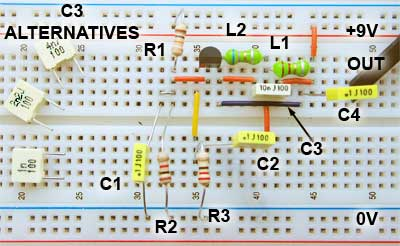# Hartley Oscillator: Working and Design using Op amp

Hartley oscillator is a LC feedback circuit used to generate high-frequency waveforms or signals into which the oscillation frequency is determined by a tank circuit comprising of two inductors and one capacitor. In our previous article, we explain oscillator circuit and oscillator types in detail. In this article, we are going to explain Hartley oscillator and its circuit in brief.This oscillator was invented by the American engineer Ralph Hartley in 1915 while he was working for the Western Electric company. For producing good quality sine wave signals in the RF range, we preferred this oscillator.

## Hartley oscillator Circuit

As shown in Figure below, in common emitter configuration NPN transistor connected serves as an active device in amplifier stage, R1 and R2 are biasing resistors, RFC is the radio frequency choke which provides the isolation between AC and DC operation, capacitors Cc1 & Cc2 are the coupling capacitors.

When the Dc supply is given to the circuit, collector current starts flowing and begins the charging of the capacitor C. When the capacitor is fully charged, it starts discharging through inductors L1 and L2 and again starts charging. To the base of the transistor, the tank circuit is coupled through the capacitor Cc1 and generates a sine wave. The transistor connected takes the input from tank circuit and converts it to a standard sine wave with a leading positive waveform. To correct and amplify the signal generated by the tank circuit, transistor provides amplification as well as inversion. From collector-emitter circuit to base-emitter circuit, the mutual inductance between the L1 and L2 provides the feedback energy.

At the frequency of oscillation, the impedance of the collector load is resistive and an increase in the base voltage causes a decrease in the collector voltage. In oscillator circuit, 180o phase change occurs in the voltage between the base and collector and 180o occur along the feedback loop. By combining the phase changes, the correct phase relationship of positive feedback for oscillations will be maintained.

## Hartley oscillator Basics:

Hartley oscillator tank circuit:

In Hartley oscillator, the tuned tank circuit i.e. LC circuit is connected between the collector and the base of a transistor amplifier. On the tuned circuit coil the emitter is connected to a tapping point as far as the oscillatory voltage is concerned.

Hartley circuit is also known as a split inductance oscillator because of the presence of the centre tapped coil i.e. it acts like a two separate coil in very close proximity with the current flowing through coil sections. As shown in figure above, the feedback part of the tuned LC tank circuit is taken from the centre tap of the inductor coil or even two separate coils in series which are parallel with a variable capacitor C. These oscillators can be operated at frequencies lower than 20 KHz, but for lower frequencies the inductor value needs to be high and it has a practical limit.

Barkhausen Criteria:

In electronics, this stability criterion is a mathematical condition to determine when a linear electronic circuit will oscillate. It is widely in the design of negative feedback circuits such as op amp and electronic oscillators to prevent them from oscillating.

If A is the gain of the amplifying element in the circuit and β(jw) is the transfer function of the feedback path then around the feedback loop of the circuit, β A is referred as a loop gain. Following conditions at which the circuit will sustain the steady state oscillations are:

• In absolute magnitude, the loop gain is equal to unity i.e. ǀ β Aǀ =1
• The phase shift around the feedback loop is zero or an integral multiple of 2∏ i.e. β A = 2∏n, n ϵ 0,1,2…

Frequency of Hartley oscillator:

The frequency of a Hartley oscillator can be expressed using the equation:

F = 1 / 2∏ √ LC

Where

C = capacitance of the capacitor in the tank circuit

L = L1 + L2, the effective series inductance of the inductors L1 & L2 in the tank circuit

If coils are winded on the single core than the mutual induction will occur between the coils which change the behaviour of the oscillator circuit. For that purpose, we assumed that both inductors coils to be winded on a single core.

### Hartley oscillator circuit using op-amp:

Hartley oscillator circuit with operational amplifier generally facilitates the gain adjustment using the feedback resistance and internal resistance.

The typical arrangement is shown above indicates that in transistorized oscillator the gain depends on the tank circuit element L1 & L2 i.e. equal to the ratio of L1 & L2 whereas in op amp oscillator the gain can be expressed by the equation A = -Rf / R1 and hence provide great frequency stability. The main advantage of using op amp is that the gain of the oscillator can be individually adjusted using the feedback resistor (Rf) and input resistor (R1). The working principle and frequency equation of the op amp type is same as that of the transistor type.

Frequency of oscillation of this type of oscillator is given as:

F = 1 / 2∏ √ Leq C

Where

Leq = L1 + L2 + 2M or L1 + L2

To generate oscillation from this circuit, amplifier gain must be selected greater than or, at least, equal to the ratio of two inductances i.e.

Av = L1 / L2

If the mutual inductance exists between L1 & L2 then the gain becomes equal to

Av = (L1+M) / (L2+M)

Following of the advantages of Hartley oscillator are:

• The output amplitude remains constant over the frequency range.
• Few components required i.e. either a tapped coil or two fixed inductors are needed.
• Easily create an accurate fixed frequency crystal oscillator.
• By using a single variable capacitor, the frequency may be adjusted.